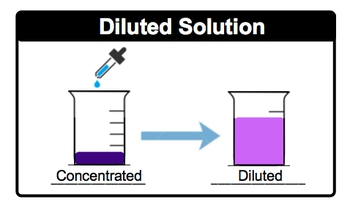## GOB Chemistry

Learn the toughest concepts covered in your GOB - General, Organic, and Biological Chemistry class with step-by-step video tutorials and practice problems.

Solutions

# Dilutions

In Dilutions, a solvent (usually water) is added to a concentrated solution.

Concentrated & Diluted Solutions
1
concept

## Dilutions1m
Play a video:
a standard, sometimes referred to as a stock solution, is a concentrated solution that will be diluted for some laboratory use later on. And we're gonna say dilution is just the addition of more solvent, usually water to a solution in order to create a lower concentration. So, if we take a look here, we have our purple solvent here, purple solution. Actually, it's pretty dark purple, meaning that it's concentrated. And what we're doing here is we're slowly adding more water, so we're adding water to it to diluted. As a result of this, it goes from being a dark purple to a lighter type of fuchsia or purple. That's showing us that it's not as concentrated as it was before. So here this represents our diluted soldier. So, just remember when we're talking about dilution, we're just talking about adding water to our original solution to make it less concentrated.2
example

## Dilutions Example 11m
Play a video:
in this example question. It says, if each sphere represents a mole of salute from the images provided below, arrange the solutions from least concentrated to most concentrated. Alright, so least concentrated is the same thing as saying the lowest polarity most concentrated means we have the highest polarity. Now remember, Polarity itself represents moles of solute divided by leaders of solution. So if we take a look here for a a has in it 12345 Sears. So that would be five moles of solute divided by one leader of solutions. So that'd be five Mueller for B b is 123 spheres. So that's three moles of solute over to leaders of solutions that be 1.5 Mueller and then finally see, we have 123456 spheres. So six moles divided by three leaders of solution. So that's two more so arranging it from the lowest polarity toe highest polarity, we're going to say the order would be be then see, and then finally a would have the highest polarity
3
concept

## Dilutions58s
Play a video:
So at this point we know that a dilution makes our solutions less concentrated. It takes us from a larger, more clarity value toe a smaller polarity value. We're gonna say dilution can be expressed by the following equation. So it's M one V one equals M two V to hear M one and V one represent the polarity and volume before dilution. While M two and V two are after the dilution, we're going to stay here. M one is before a solvent has added So m one which is the more concentrated solution is always larger than M two, which will be the diluted solution. Now the two represents your final volume. And how exactly did we get to be too? Well, we started out with an initial volume and we added water to it. So the two equals the one plus the volume of solvent added4
example

## Dilutions Example 22m
Play a video:
5
Problem

To what final volume would 100 mL of 5.0 M KCl have to be diluted in order to make a solution that is 0.54 M KCl?

6
Problem

If 880 mL of water is added to 125.0 mL of a 0.770 M HBrO4 solution what is the resulting molarity?

7
Problem

A student prepared a stock solution by dissolving 25.00 g of NaOH in enough water to make 150.0 mL solution. The student took 20.0 mL of the stock solution and diluted it with enough water to make 250.0 mL solution. Finally taking 75.0 mL of that solution and dissolving it in water to make 500 mL solution. What is the concentration of NaOH for this final solution? (MW of NaOH:40.00 g/mol).